# Chart representation

Snow Flower Text supports drawing of more than 10 types of charts. In the AsciiDoc document, the template is inserted by selecting the chart type from the menu, and the chart can be easily embedded in the document simply by editing the CSV format data. Charts provide a visual representation of the characteristics of the data and are useful for getting a general overview of the data. By embedding charts in documents, anyone can easily create compelling reports.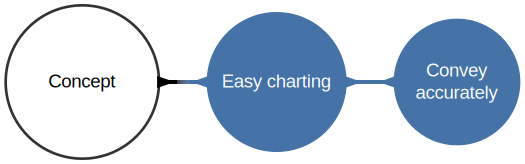The chart function is an extension of Snow Flower Text’s original AsciiDoc. This HTML document itself is also created using Snow Flower Text.

## 1. Features

The chart function of Snow Flower Text has the following features.

Searchable

Labels on rendered charts remain text searchable. This feature is inherited after exporting to formats such as HTML, PDF, and DocBook. It has better Findability than embedding raster images.

Change Trackable

Chart information is described in highly readable text. Thus, changes made in multiple versions can be easily found by comparing common texts. This is an excellent feature not found in office software that stores information in binary. Superior traceability compared to binary format files.

Vector image

Charts are vector images, so they will render sharp and clean on any medium. No jaggies occur, no matter how large they are. It has better portability than embedding raster images.

Universal color

The color palette used to color the charts uses a color universal design so that everyone can see the colors easily.

## 2. Chart classification

Snow Flower Text supports more than 10 chart types. Charts are classified by plotting method. Specifiable parameters and data description methods differ depending on the chart classification.

Category Plot

A category plot is a two-dimensional plot of a continuous numeric value on one axis and a non-numeric (string) category on the other axis. The following charts belong to this category:

• Bar graph (bar)

• Stacked-bar (stacked-bar)

• Line graph (line)

• Area chart (area)

• Stacked-area chart (stacked-area)

XY plot

An XY plot is a two-dimensional plot of continuous numerical values on two axes. The following charts belong to this category:

• XY graph (xy)

• Histogram

• Scatter chart

Pie plot

This is a circular plot. This category includes pie charts and their variants.

• Pie chart (pie)

• Ring graph (ring)

## 3. How to use

Charts can be embedded in documents by writing CSV format text data in the `[chart]` block of AsciiDoc.

1. Select the AsciiDoc grammar (`Edit` > `Select grammer..` > `AsciiDoc`).

2. Use the `[chart]` block to embed a chart. Insert the chart template into the document by operating the `Menu` > `Insert` > `Chart…​` menu selection.

3. Describe the data in CSV format. For details, refer to the following “How to write a chart block”.

## 4. How to write a chart block

By using the following syntax in the AsciiDoc document, you can embed the chart in the document at that position.

``[chart, Type of Chart, List of chart parameters]--------------------Data notation--------------------``

For example, a simple bar chart can be written as follows

Example
``[chart, bar, categoryLabel="category",valueLabel="value"]-------------------------------------A,  10B,  20C,  30-------------------------------------``

This example is rendered as: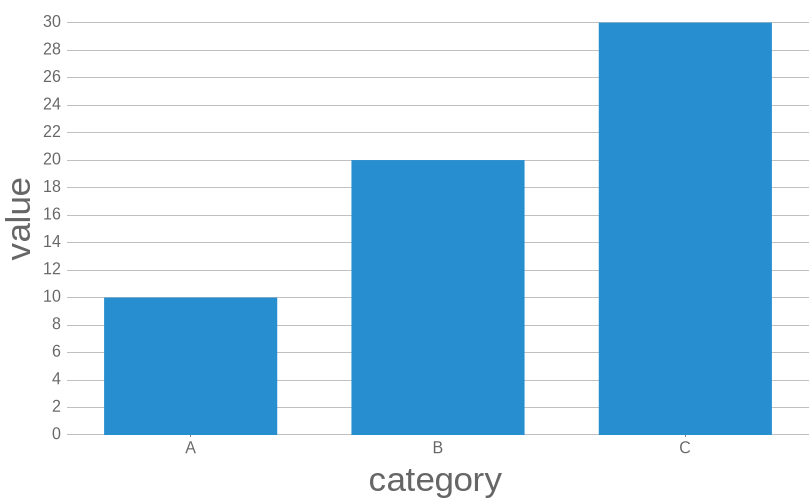### 4.1. Type of Chart

The type of the chart is specified in the second parameter of the chart block. Refer to the following table for the parameters corresponding to the chart type.

Table 1. Define a parameter to specify the chart type
Parameter 　Description

`bar`

Bar chart

`stacked-bar`

Stacked bar chart

`line`

Line chart

`area`

Area chart

`stacked-area`

Stacked area chart

`radar`

`xy`

XY chart

`histogram`

histogram

`scatter`

Scatter plot

`pie`

Pie chart

`ring`

Doughnut chart

For example, if you want to embed a bar chart, specify `bar` as the second parameter of the `[chart]` block as follows

``[chart, bar]``
Bar chart

A bar chart is a graph that expresses some value by the length of a rectangular bar. Bar charts are used to compare two or more values. There are vertical and horizontal directions for the bar to extend. Bars are spaced in the Bar chart, but not in the histogram.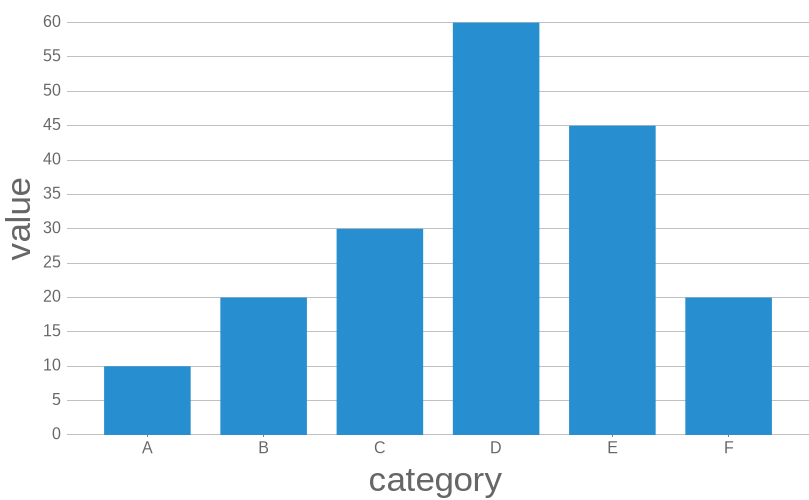`[chart,bar, …​]`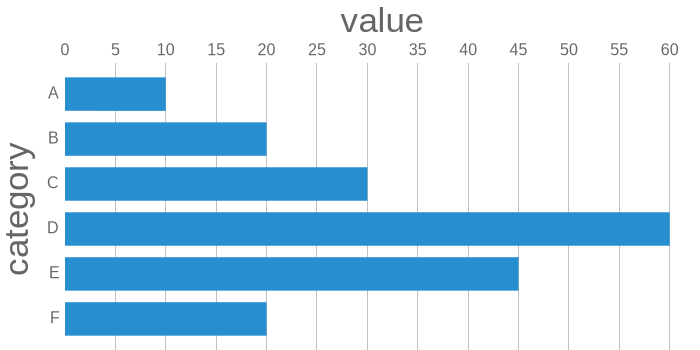`[chart, bar, orientation=horizontal, …​]`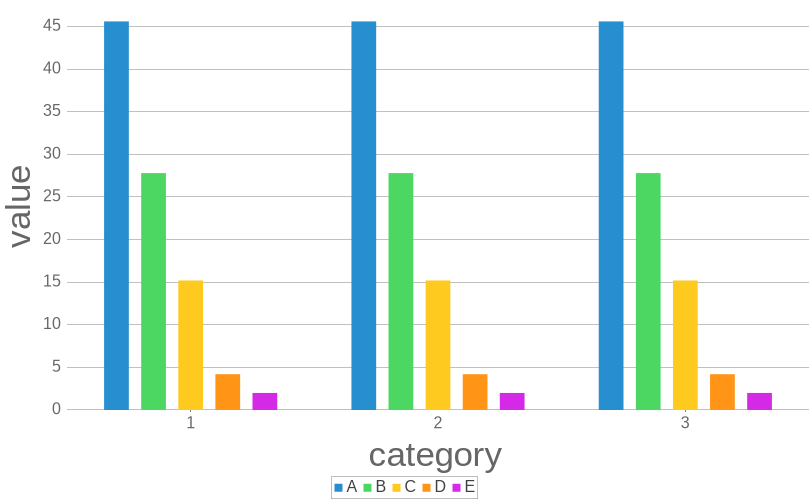`[chart, bar,…​]`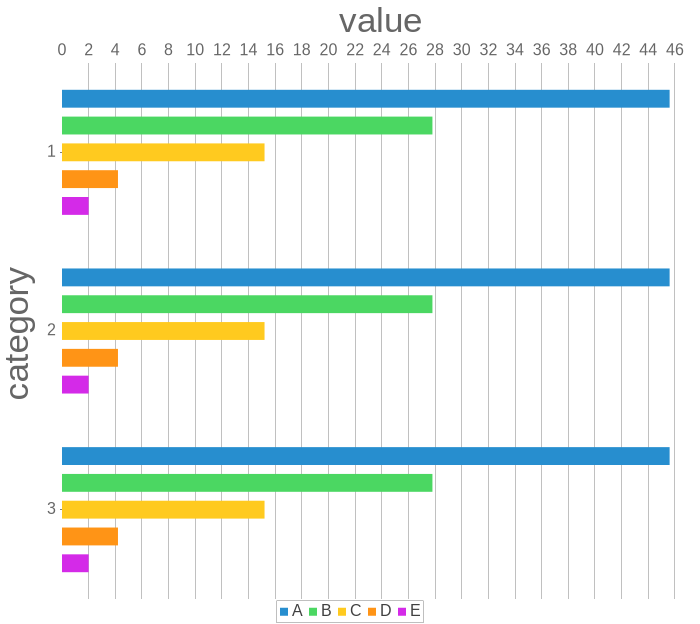`[chart, bar, orientation=horizontal, …​]`
Stacked-bar chart

A stacked bar chart is a type of bar chart, and is a graph expressed by stacking elements in the same item on top. In the stacking bar chart, each element in the item is represented in a different color, which is suitable for grasping the total amount of each item and the ratio of each element in the item. For example, displaying the stacking bar chart for each day of the week or year makes it easier to understand the day of the week transition and annual transition of the element.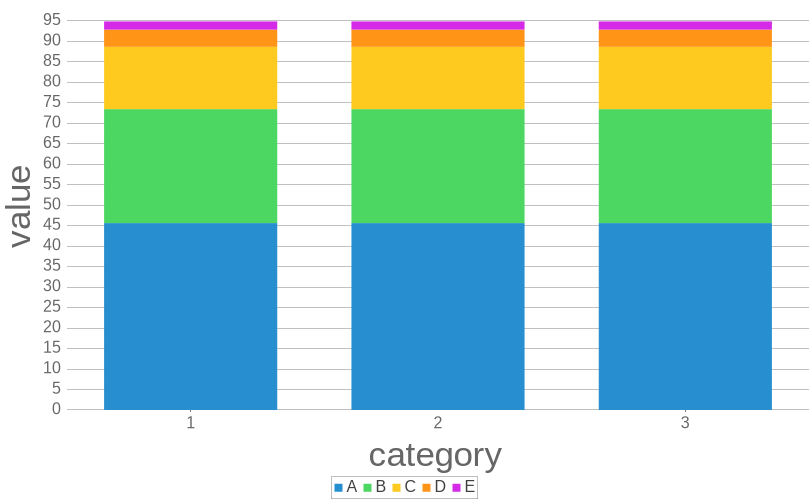`[chart, stacked-bar,…​]`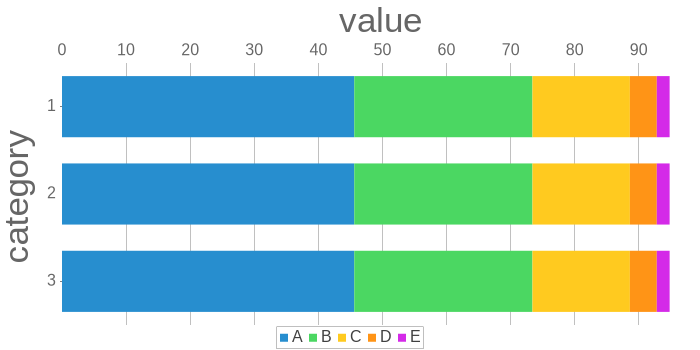`[chart, stacked-bar, orientation=horizontal,…​]`
Line chart

A line chart is a straight line connecting the plotted points. It is suitable for grasping the transition of data over a certain period of time.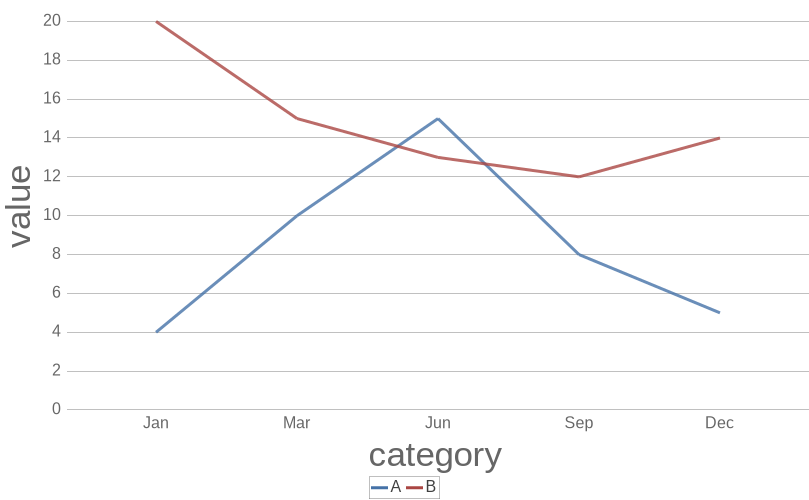`[chart, line,…​]`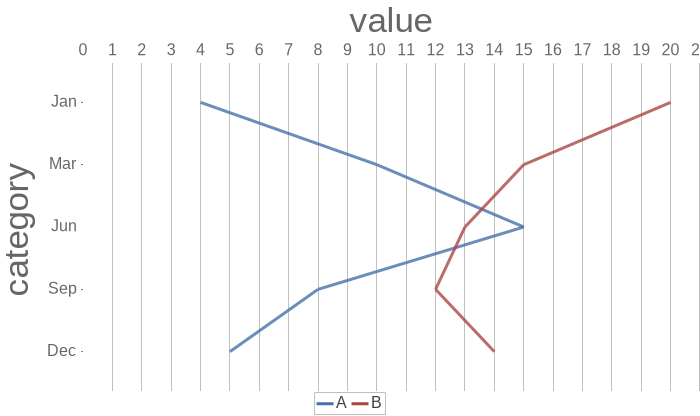`[chart, line, orientation=horizontal,…​]`
Area chart

Area chart is a type of line chart that displays quantitative data. Generally, two or more series of data groups are compared in a graph, and the area between the axis and the line is highlighted by color.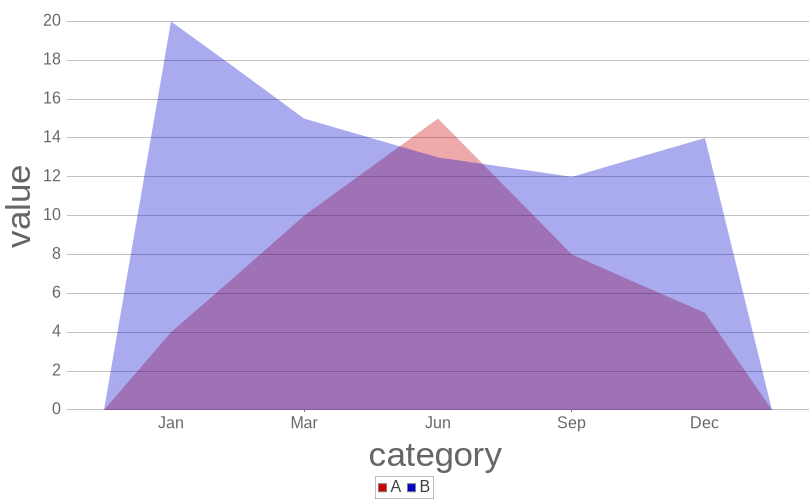`[chart, area,…​]`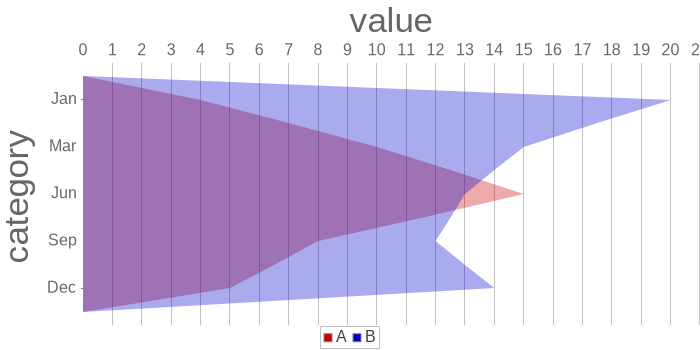`[chart, area, orientation=horizontal,…​]`
Stacked-area chart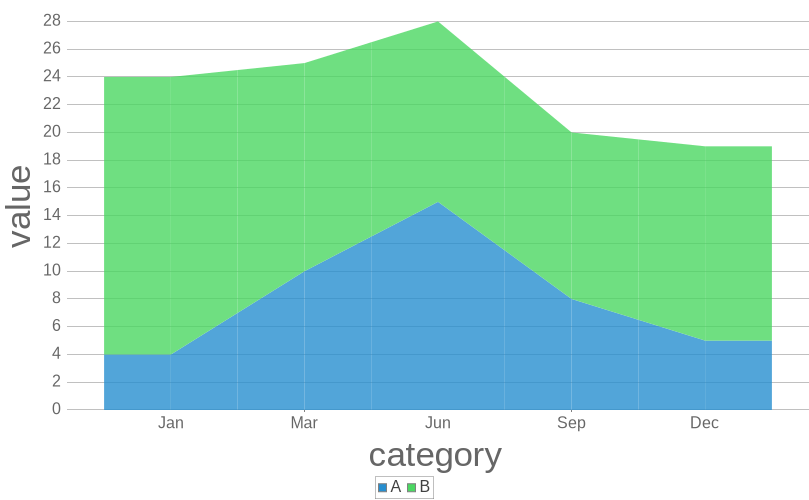`[chart,stacked-area,…​]`

A radar chart is a diagram in which multiple items are set on the vertical axis and the origins on the vertical axis are combined into a single radial chart. You can compare the sizes of multiple items at a glance. Also known as a spider web chart.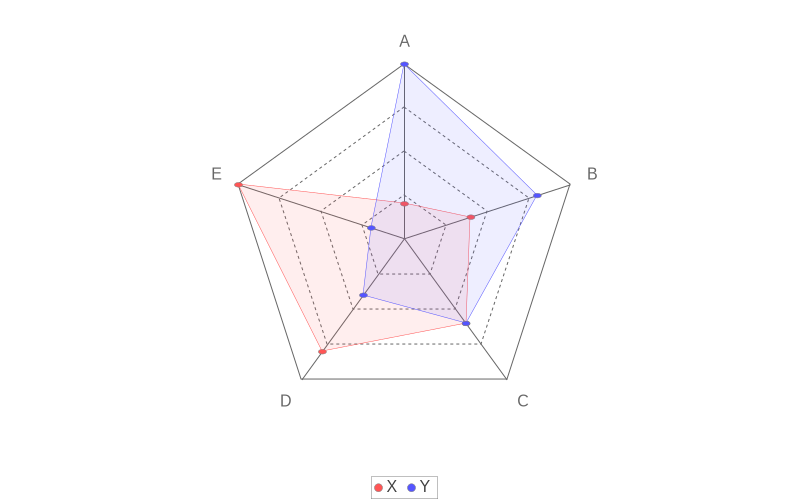`[chart, radar, grid=true,…​]`
Scatter plot

The scatter plot is a plot of data with dots corresponding to the amount and size of two items on the vertical and horizontal axes. The scatter plot has the feature of being able to grasp the distribution and correlation of the two items. If the data group tends to be distributed upward to the right, there is a positive correlation, and if the data group tends to be distributed downward to the right, there is a negative correlation. Scatter plots are often used to display and compare numerical data such as scientific, statistical, and engineering data.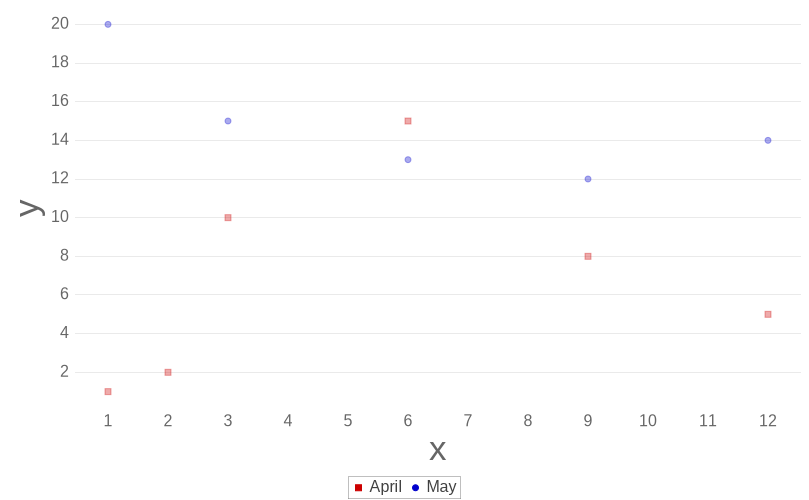`[chart, scatter,…​]`
XY char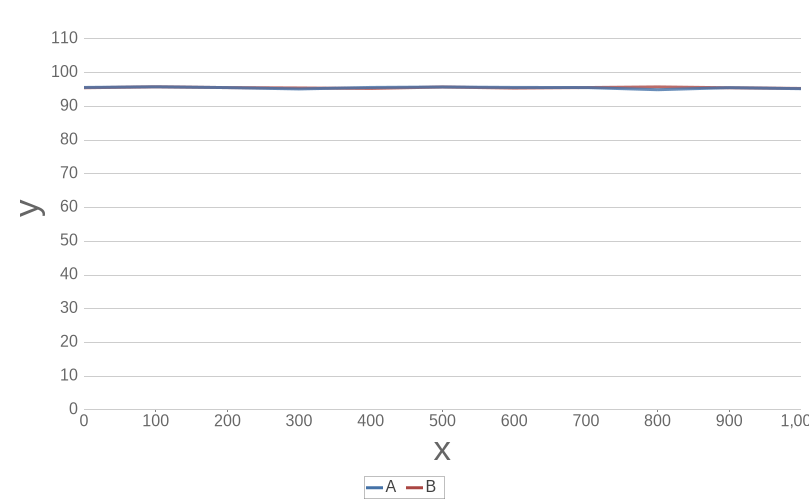`[chart, xy,…​]`
Histogram

A histogram is a type of statistical graph with frequencies on the vertical axis and classes on the horizontal axis. It is mainly used in statistics, mathematics, image processing, etc. to visually recognize the distribution of data. In the industrial field, it is known as the seven QC tools for quality control. In the histogram he specifies the number of bins as a parameter.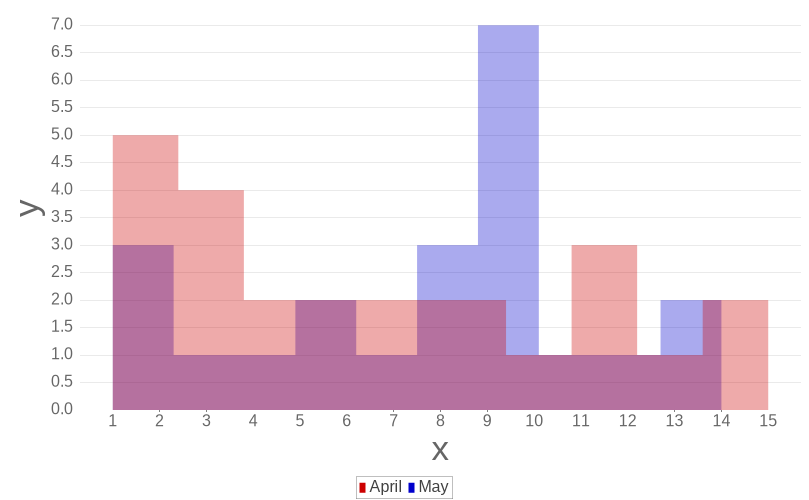`[chart, histogram, bins=10,…​]`
Pie chart

A pie chart is a graph in which a circle is the entire data (100%) and the composition ratio of the elements in it is represented by the area of a sector. In a pie chart, the length of a sector arc is proportional to the quantity represented by that sector. Some statisticians recommend not using pie charts because it is difficult to compare the size of individual regions compared to bar charts and the like.

Pie charts ignore negative values in the data.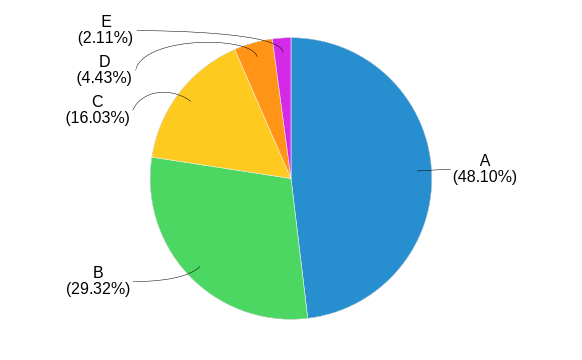`[chart,pie]`
Doughnut chart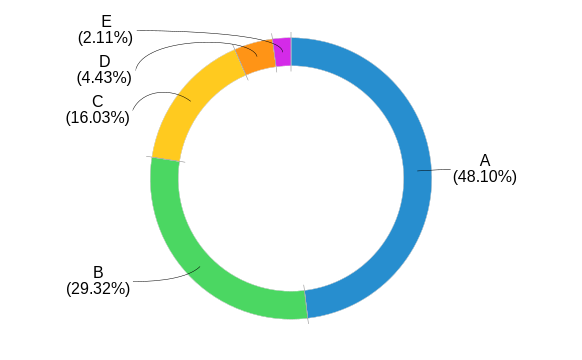`[chart,ring]`

### 4.2. Chart block parameters

The block parameters that can be used differ depending on the chart type. Refer to the following table for the combinations of chart types and parameters that can be used.

Table 2. Parameters available for the ‘chart’ block (1)
widthfontSizerotateorientation

bar

Yes

Yes

Yes

Yes

stacked-bar

Yes

Yes

Yes

Yes

line

Yes

Yes

Yes

Yes

area

Yes

Yes

Yes

Yes

stacked-area

Yes

Yes

Yes

xy

Yes

Yes

Yes

scatter

Yes

Yes

Yes

histogram

Yes

Yes

Yes

pie

Yes

Yes

ring

Yes

Yes

Yes

Yes

Table 3. Parameters available for the ‘chart’ block (2)
captionxLabelyLabelcategoryLabelvalueLabel

bar

Yes

Yes

Yes

stacked-bar

Yes

Yes

Yes

line

Yes

Yes

Yes

area

Yes

Yes

Yes

stacked-area

Yes

Yes

Yes

xy

Yes

Yes

Yes

scatter

Yes

Yes

Yes

histogram

Yes

Yes

Yes

pie

Yes

ring

Yes

Yes

Table 4. Parameters available for the ‘chart’ block (3)
binsgrid

bar

stacked-bar

line

area

stacked-area

xy

scatter

histogram

Yes

pie

ring

Yes

#### 4.2.1. Parameter definition

`width`

Chart width(75%, )

When displaying at 75% of the page width
``width=75%``
When displaying at 400 pixels
``width=400``
`rotate`

Rotate the category label. Specify by degrees.

To rotate 45 degrees
``rotate=45``
To rotate 90 degrees
``rotate=90``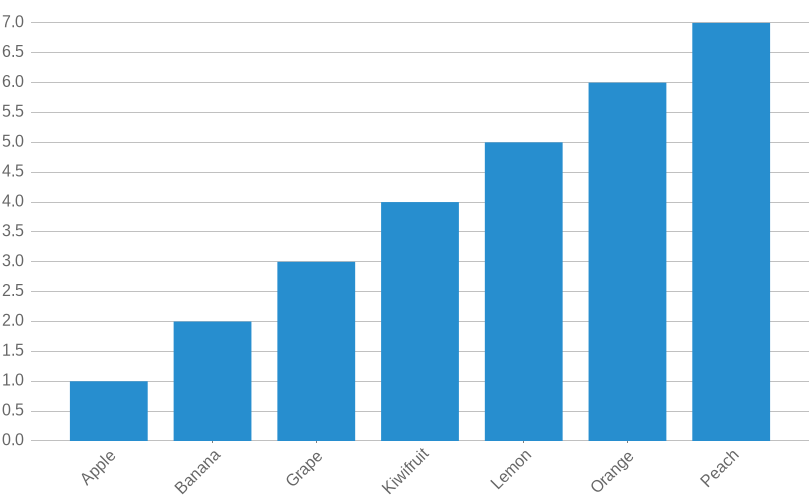`rotate=45`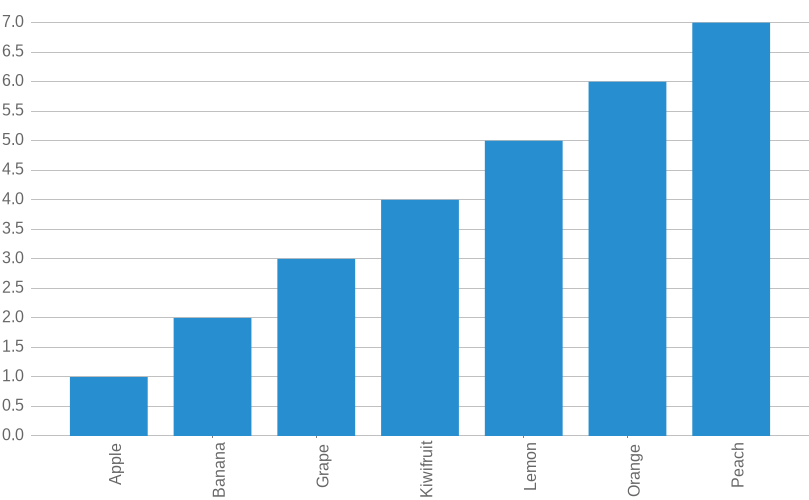`rotate=90`
`fontSize`

Font size ratio (0 or greater). If you want to increase the font size, specify a value greater than 1. Specifying the font size affects all labels.

When increasing the font size by 1.8 times
``fontSize=1.8``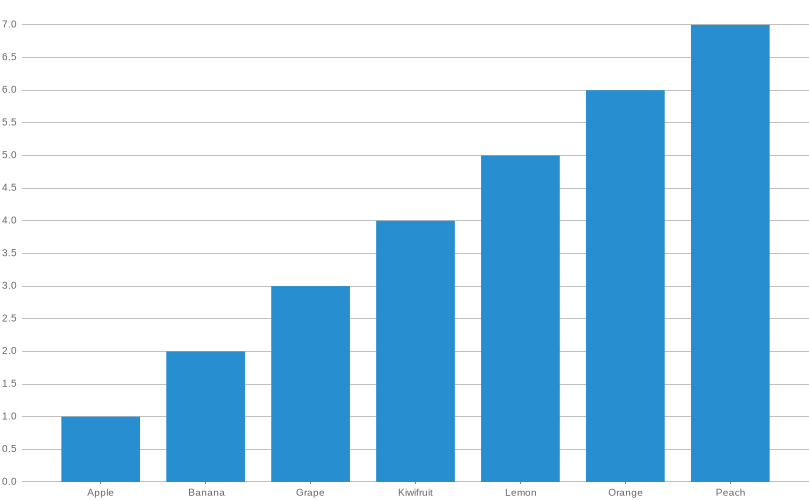`fontSize=1`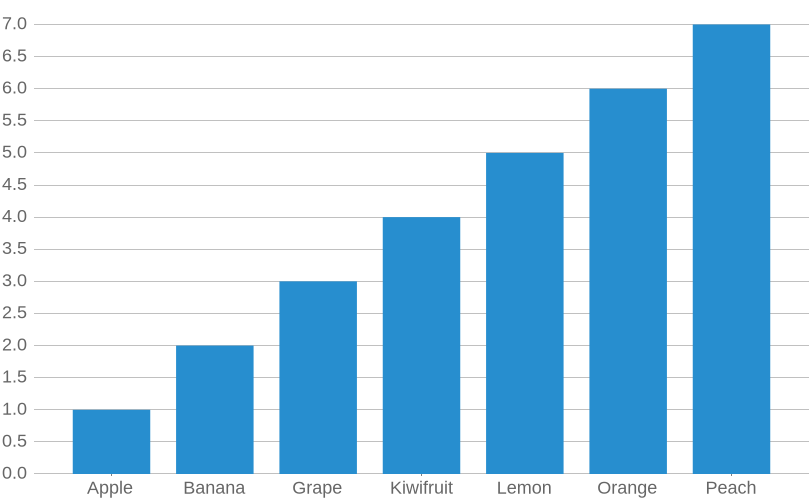`fontSize=1.8`
`orientation`

Specifies the direction of the plot. You can set `vertical` or `horizontal`. The default is `vertical`.

When plotting vertically
``orientaion=vertical``
When plotting horizontally
``orientaion=horizontal``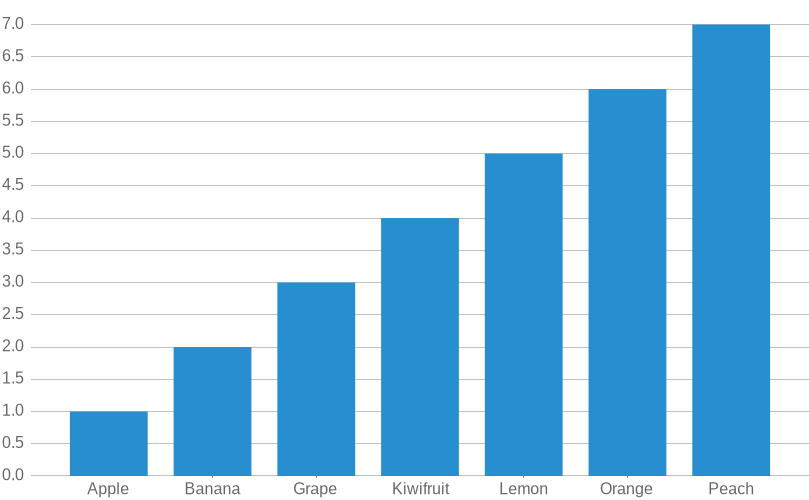`orientation=vertical`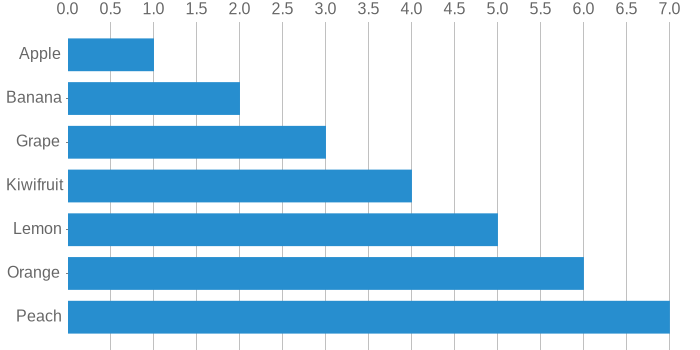`orientation=horizontal`
`caption`

Chart caption

`xLabel`

X-axis label

`yLabel`

Y-axis label

`categoryLabel`

Category label

`valueLabel`

Value label

`grid`

This parameter controls the grid display. You can specify true or false. If not specified, it will be false and the grid will be hidden.

When hiding the grid
``grid=false``
When displaying the grid
``grid=false``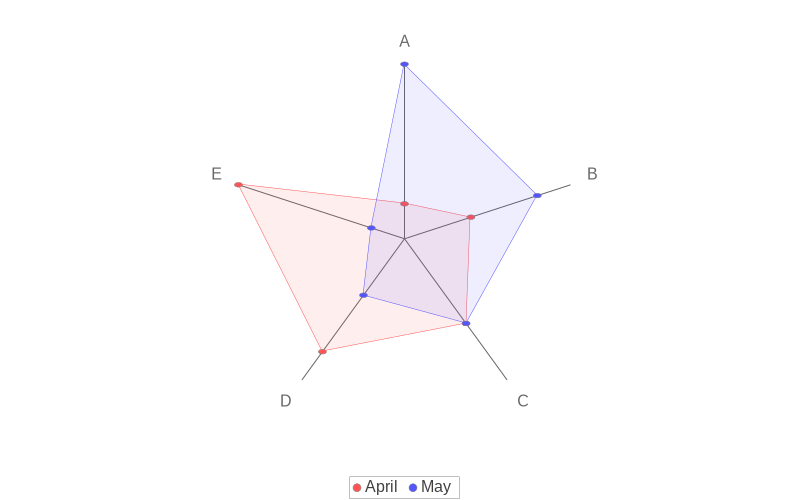`grid=false`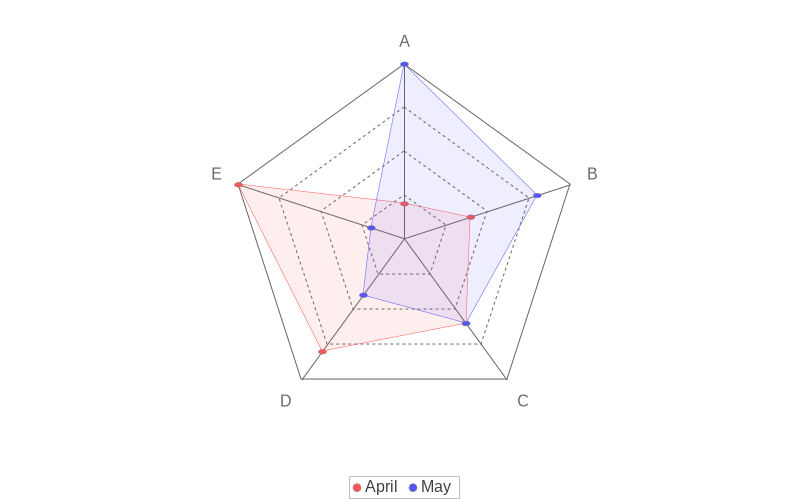`grid=true`
`bins`

A histogram-specific parameter that specifies the number of bins.

When setting the number of bins to 5
``bins=5``
When setting the number of bins to 10
``bins=10``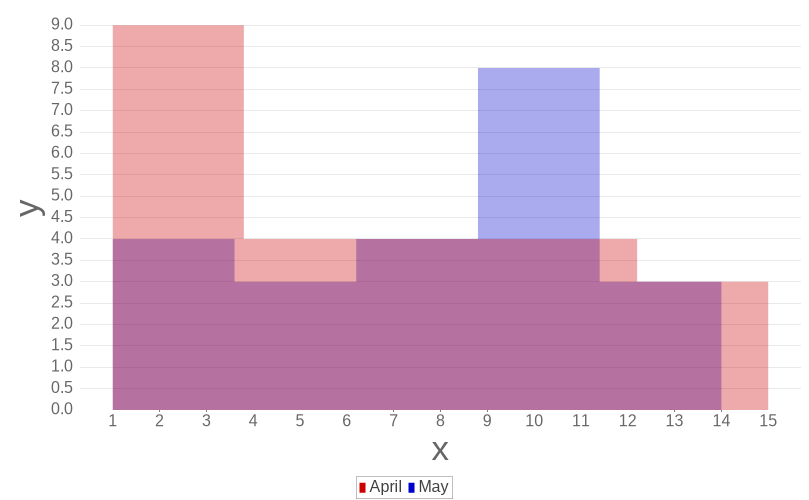`[chart, histogram, bins=5]``[chart, histogram, bins=10]`

### 4.3. How to describe the data

The description format of chart data differs depending on the chart type classification.

Category plot

Charts classified as category plots describe data in the following CSV format. If there is only one series, the series name can be omitted.

``(//series-name)?(category-name, numerical-value)+``

If you want to specify multiple series, specify the series name.

``[chart, bar]--------------------------------//Series-ACategory1,1Category2,2//Series-BCategory1,1Category2,2--------------------------------``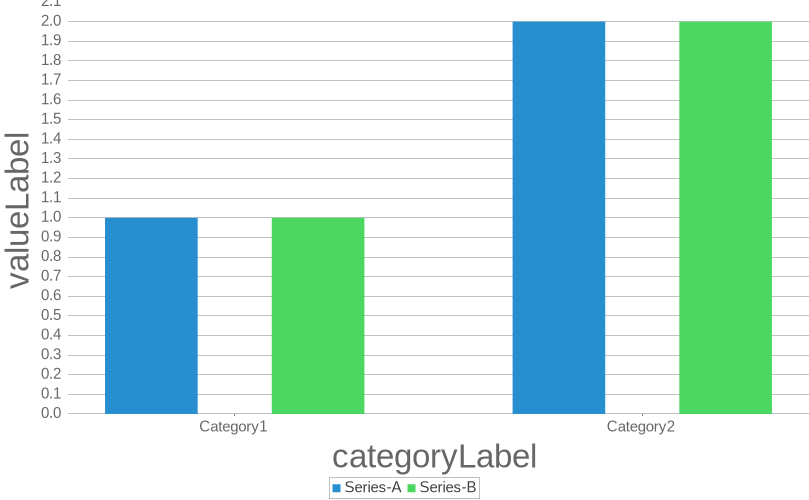XY Plots

Charts classified as XY plots describe data in the following CSV format. If there is only one series, the series name can be omitted.

``(//Series-name)?(X-value, Y-value)+``

If you want to specify multiple series, specify the series name.

``[chart, xy]--------------------------------//SeriesA1,12,2//SeriesB1,1.52,2.5--------------------------------``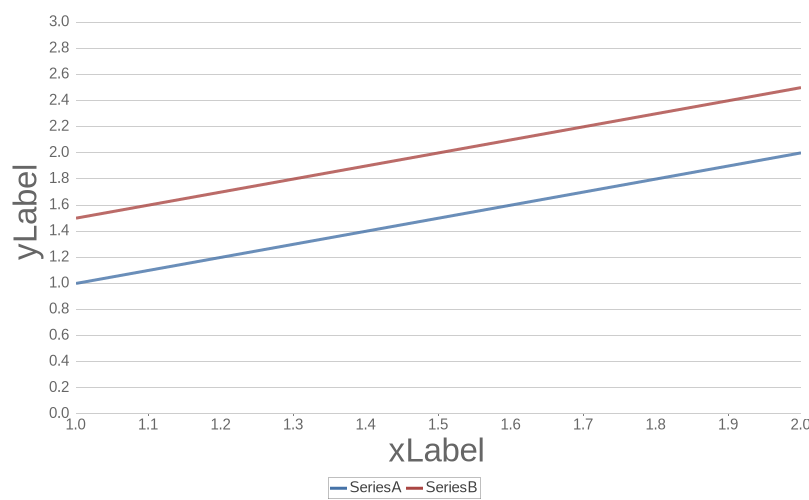Pie chart

Charts classified as Pie plot describe data in the following CSV format.

``(Caregory-name, numerical-value)+``

If you want to specify multiple series, specify the series name.

``[chart, pie]--------------------------------Category1,1Category2,2--------------------------------``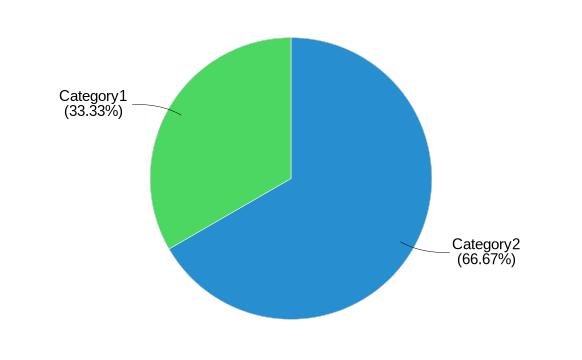## 5. Sample source

Here are some complete sample sources for chart output. Please refer to them when editing the chart.

### 5.1. Bar chart

``[chart,bar, categoryLabel="category",valueLabel="value"]-------------------------------------//A1,  45.62,  45.63,  45.6//B1,  27.82,  27.83,  27.8//C1,  15.22,  15.23,  15.2//D1,  4.22,  4.23,  4.2//E1, 22, 23, 2-------------------------------------````[chart,bar, orientation=horizontal, categoryLabel="category",valueLabel="value"]-------------------------------------1, 102, 203, 304, 605, 456, 20-------------------------------------``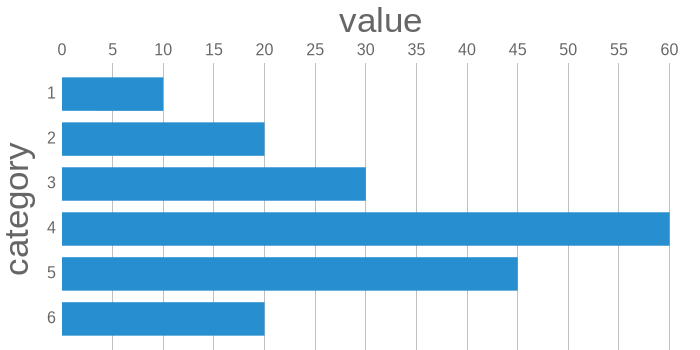``[chart,bar, orientation=horizontal, categoryLabel="category",valueLabel="value"]-------------------------------------//A1,  45.62,  45.63,  45.6//B1,  27.82,  27.83,  27.8//C1,  15.22,  15.23,  15.2//D1,  4.22,  4.23,  4.2//E1, 22, 23, 2-------------------------------------``### 5.2. Stacked bar chart

``.`[chart,stacked-bar,...]`[chart,stacked-bar,categoryLabel="category",valueLabel="value"]-------------------------------------//A1,  45.62,  45.63,  45.6//B1,  27.82,  27.83,  27.8//C1,  15.22,  15.23,  15.2//D1,  4.22,  4.23,  4.2//E1, 22, 23, 2-------------------------------------```[chart,stacked-bar,…​]`
``[chart,stacked-bar,orientation=horizontal, categoryLabel="category",valueLabel="value"]-------------------------------------//A1,  45.62,  45.63,  45.6//B1,  27.82,  27.83,  27.8//C1,  15.22,  15.23,  15.2//D1,  4.22,  4.23,  4.2//E1, 22, 23, 2-------------------------------------``### 5.3. Line chart

``[chart,line,categoryLabel="category",valueLabel="value"]-------------------------------------//1x1,  12,  23,  34,  45,  56,  67,  7//4x1,  42,  83,  124,  165,  206,  247,  28//4^21,  12,  43,  94,  165,  256,  367,  49-------------------------------------``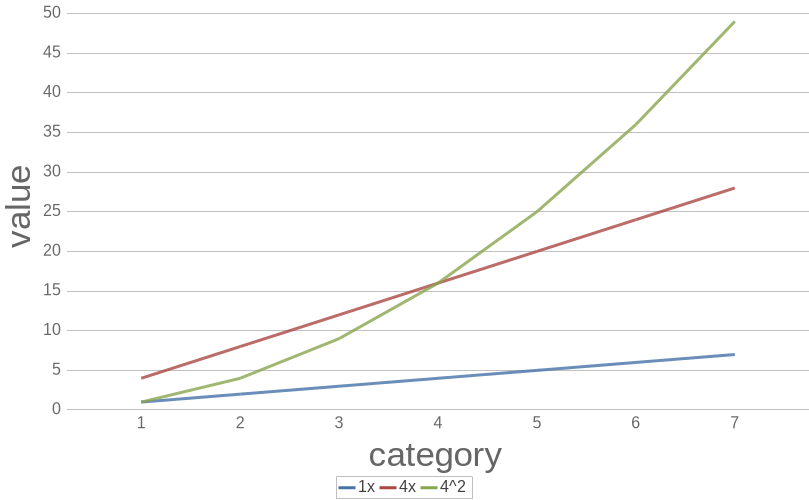### 5.4. Area chart

``[chart,area, title="Area chart",categoryLabel="category",valueLabel="value"]-------------------------------------//April1,  43,  106,  159,  812, 5//May1,  203,  156,  139,  1212, 14-------------------------------------``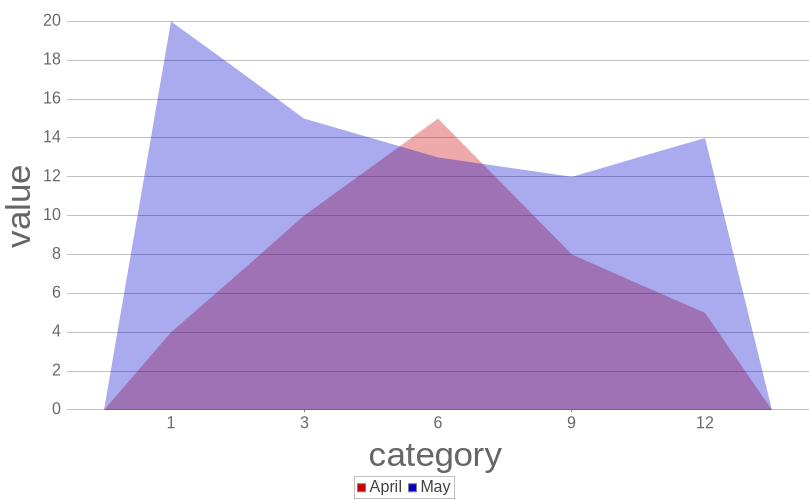Area chart
``[chart,area, title="Area chart", orientation=horizontal, categoryLabel="category",valueLabel="value"]-------------------------------------//April1,  43,  106,  159,  812, 5//May1,  203,  156,  139,  1212, 14-------------------------------------``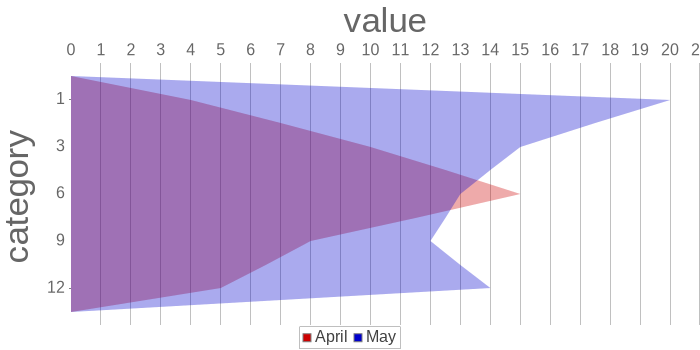### 5.5. Stacked area chart

``[chart,stacked-area,categoryLabel="category",valueLabel="value"]-------------------------------------//April1,  43,  106,  159,  812, 5//May1,  203,  156,  139,  1212, 14-------------------------------------``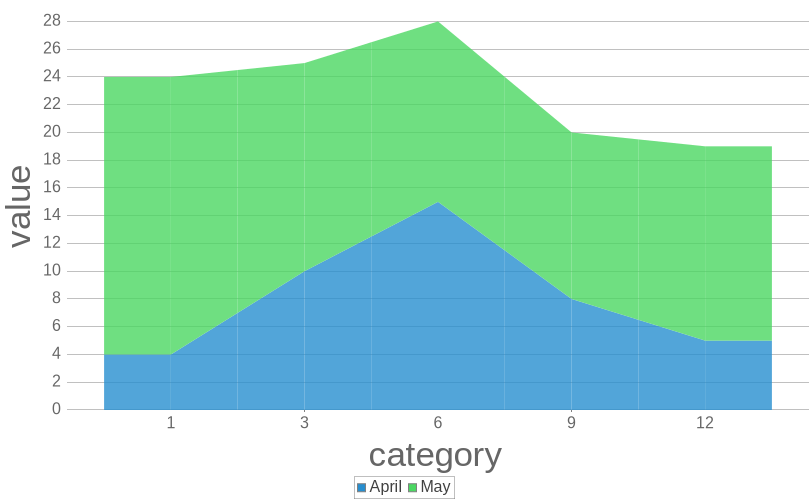### 5.6. XY chart

``[chart,xy,xLabel="x",yLabel="y"]-------------------------------------// A0,95.599100,95.785200,95.550300,95.145400,95.598500,95.729600,95.600700,95.564800,94.919900,95.5331000,95.224// B0,95.499100,95.785200,95.550300,95.445400,95.298500,95.729600,95.400700,95.564800,95.756900,95.5001000,95.30-------------------------------------``### 5.7. Scatter plot

``[chart,scatter,xLabel="x",yLabel="y"]-------------------------------------//April1,  43,  106,  159,  812, 5//May1,  203,  156,  139,  1212, 14-------------------------------------``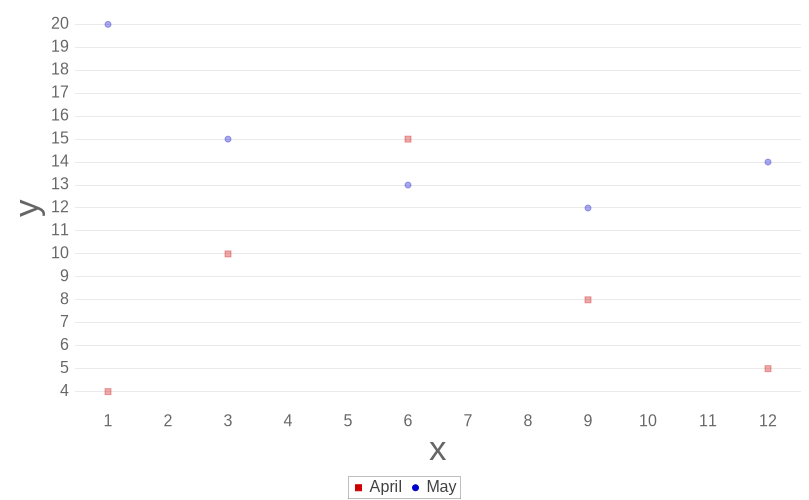### 5.8. Histgram chart

``[chart, histogram, bins=10,xLabel="x",yLabel="y"]-------------------------------------//April111123333456677.88.2910111212131415//May112345677.88.289991010101011121314-------------------------------------``### 5.9. Pie chart

``[chart, pie]--------------------------------category1,1category2,2--------------------------------``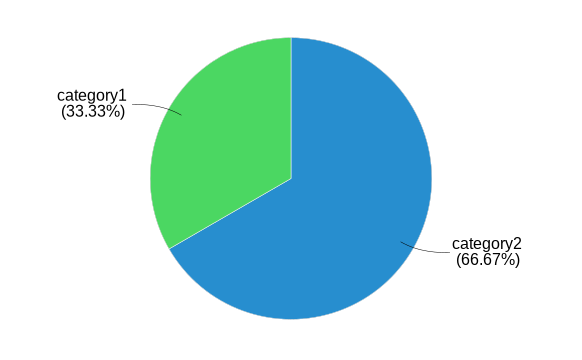### 5.10. Doughnut chart

``[chart, ring]--------------------------------category1,1category2,2--------------------------------``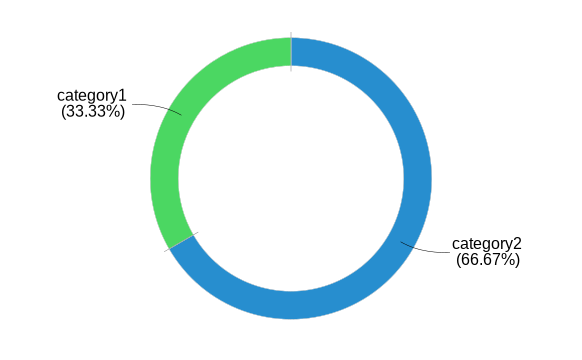``````[chart, ring, caption="Ring Chart"]
--------------------------------
category1,1
category2,2
--------------------------------``````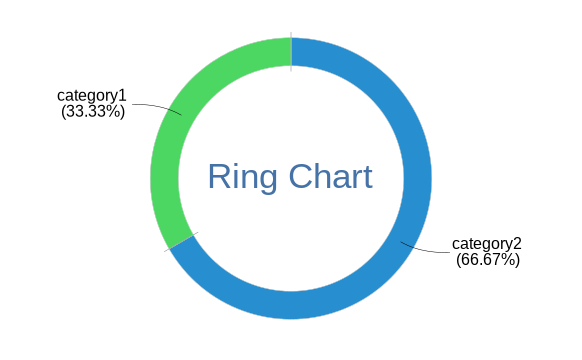``[chart,radar]-------------------------------------// XA, 1B, 2C, 3D, 4E, 5// YA, 5B, 4C, 3D, 2E, 1-------------------------------------``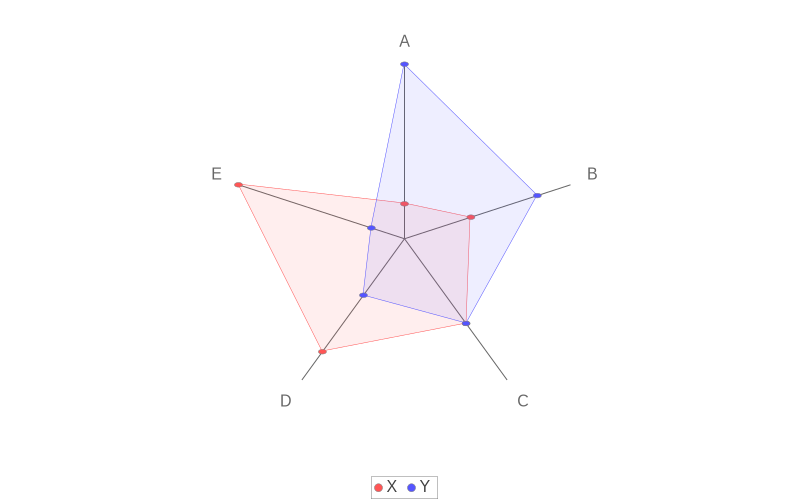``[chart,radar, fontSize="1.6", grid=true]-------------------------------------// XA, 1B, 2C, 3D, 4E, 5// YA, 5B, 4C, 3D, 2E, 1-------------------------------------``